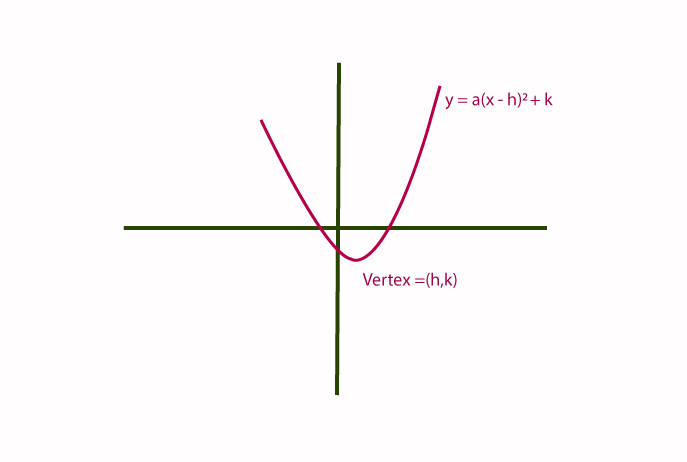# Vertex Formula

In Mathematics, the vertex formula helps to find the vertex coordinate of a parabola, when the graph crosses its axes of symmetry. Generally, the vertex point is represented by (h, k). We know that the standard equation of a parabola is y=ax2+bx+c. Here, if the coefficient of x2 is positive, the vertex should be at the bottom of the U-shaped curve. If the coefficient of x2 is negative, then the vertex should be at the top of the U-shaped curve. In this article, we are going to learn the standard form and vertex form of a parabola, vertex formula, and examples in detail.

## Vertex Form of ParabolaWe know that the standard form of the parabola is y=ax2+bx+c.

Thus, the vertex form of a parabola is y = a(x-h)2 + k.

Now, let us discuss the vertex formula in detail.

### Vertex Formula

The vertex formula is used to find the vertex of a parabola. There are two ways to find the vertex of a parabola.

Vertex, (h, k) = (-b/2a, -D/4a)

Where “D” is the discriminant where D = b2 – 4ac.

“h” and “k” are the coordinates of the vertex.

The above formula can also be written as follows:

$$\begin{array}{l}Vertex=\left(h,\:k\right)=\left(\frac{-b}{2a},c-\frac{b^{2}}{4a}\right)\end{array}$$

The other method to find the vertex of a parabola is as follows:

We know that the x-coordinate of a vertex, (i.e) h is -b/2a.

Now, substitute the x-coordinate value in the given standard form of the parabola equation y=ax2+bx+c, we will get the y-coordinate of a vertex.

### Solved Examples Using Vertex Formula

Example 1:

Find the vertex of a parabola, y=3x2+12x-12.

Solution:

Given parabola equation: y=3x2+12x-12.

The given parabola equation is of the standard form y=ax2+bx+c.

By comparing the given equation and standard form, we get

a = 3. b= 12, c = -12.

We know that the vertex formula is (-b/2a, -D/4a)

We know that D = b2 – 4ac.

Therefore, D = (12)2-4(3)(-12)

D = 144+144

D = 288.

Now, substitute all the known values in the formula, we get

Vertex, (h, k) = ( (-12/2(3)), (-288/4(3))

(h, k) = (-12/6, -288/12)

(h. k) = (-2, -24)

The vertex (h, k) of the parabola y=3x2+12x-12 is (-2, -24).

Example 2:

Find the vertex of the parabola y=3x2-6x+1.

Solution:

Given parabola equation: y = 3x2-6x+1.

The standard form of a parabola is  y=ax2+bx+c.

By comparing standard form and given parabola equations, we get a = 3, b=-6, c = 1.

We know that the formula to calculate the x-coordinate of a vertex is -b/2a.

Hence, h = -(-6)/2(3)

h = 6/6 = 1

Therefore, the x-coordinate of a vertex is 1.

Now, we need to find the y-coordinate of a vertex. (i.e.) k.

To get the value of the y-coordinate, substitute x =1 in the given equation y = 3x2-6x+1.

Hence, y-coordinate (k) = 3(1)2-6(1)+1

y-coordinate (k) = 3-6+1 = -2.

Hence, the coordinate of the vertex of a parabola (h, k) is (1, -2).

Stay tuned to BYJU’S – The Learning App for more formulas.

## Frequently Asked Questions on Vertex Formula

Q1

### What is the standard form of a parabola?

The standard form of a parabola is y=ax2+bx+c.

Q2

### What is the vertex form of a parabola?

The vertex form of a parabola is y = a(x-h)2 + k.

Q3

### What is the vertex formula?

The vertex formula is used to find the vertex of a parabola. The formula to find the vertex is (h, k) = (-b/2a, -D/4a), where D = b2-4ac.